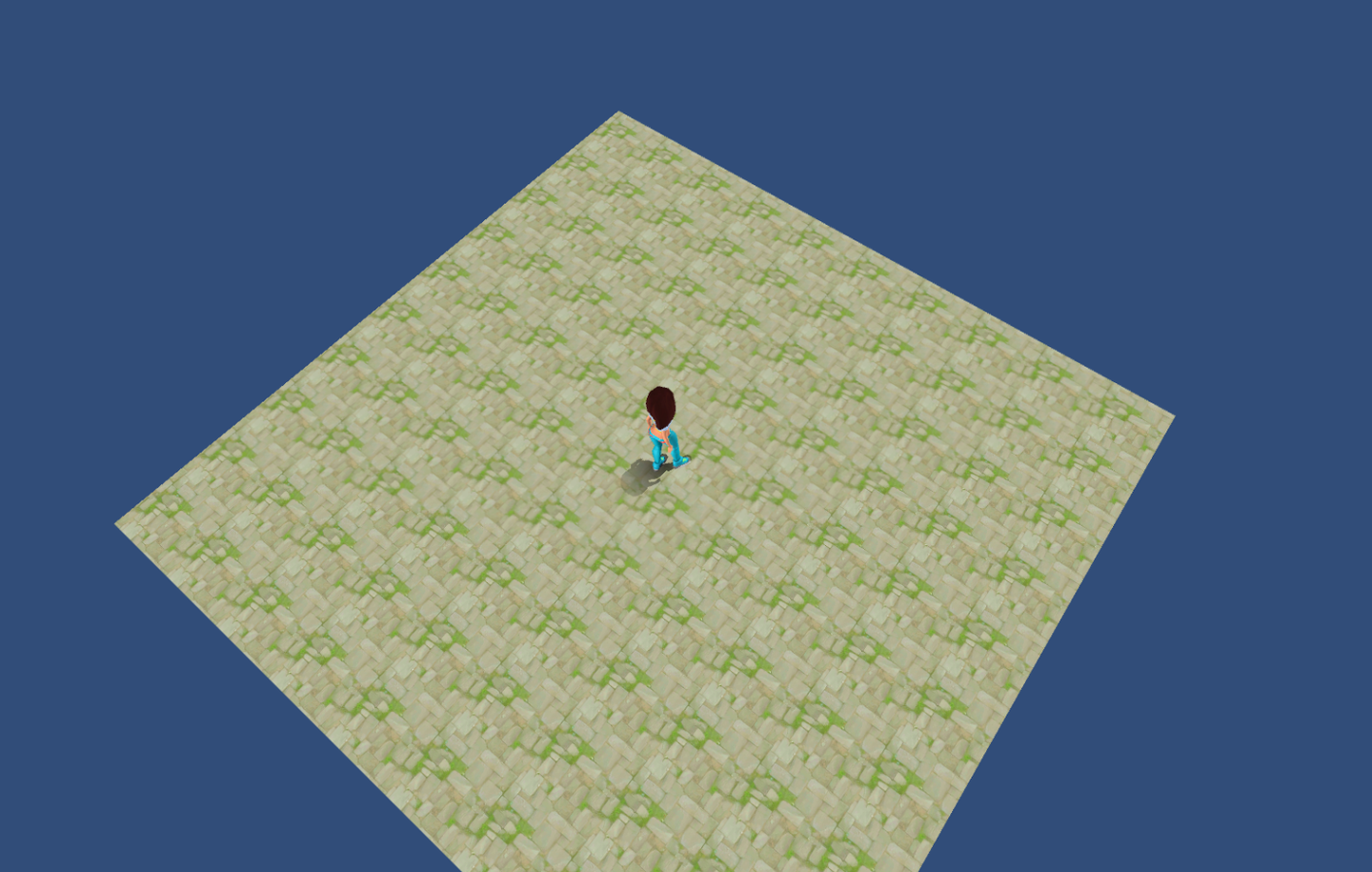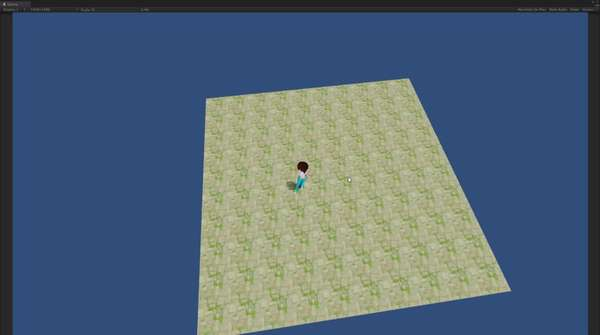## 前言https://www.zhihu.com/video/1016815623216791552

## 分析

attribute vec4 _glesVertex;
uniform highp mat4 _Object2World;
uniform highp mat4 unity_MatrixVP;
uniform highp vec4 _WorldPos;
varying highp vec3 xlv_TEXCOORD0;
varying highp vec3 xlv_TEXCOORD1;
void main ()
{
highp vec4 tmpvar_1;
highp vec3 tmpvar_2;
tmpvar_2 = (_Object2World * _glesVertex).xyz;
highp vec3 tmpvar_3;
tmpvar_3 = (tmpvar_2 - ((
/
) * tmpvar_1.xyz));
highp vec4 tmpvar_4;
tmpvar_4.w = 1.0;
tmpvar_4.xyz = tmpvar_3;
gl_Position = (unity_MatrixVP * tmpvar_4);
xlv_TEXCOORD0 = _WorldPos.xyz;
xlv_TEXCOORD1 = tmpvar_3;
}
precision highp float;
varying highp vec3 xlv_TEXCOORD0;
varying highp vec3 xlv_TEXCOORD1;
void main ()
{
lowp vec4 tmpvar_1;
mediump vec3 posToPlane_2;
highp vec3 tmpvar_3;
tmpvar_3 = (xlv_TEXCOORD0 - xlv_TEXCOORD1);
posToPlane_2 = tmpvar_3;
mediump vec4 tmpvar_4;
tmpvar_4.xyz = vec3(0.0, 0.0, 0.0);
tmpvar_4.w = (pow ((1.0 -
clamp (((sqrt(
dot (posToPlane_2, posToPlane_2)
tmpvar_1 = tmpvar_4;
gl_FragData = tmpvar_1;
}
• 几个需要用到的寄存器状态的数值

WorldPos 人物的当前坐标

ShadowFaceParams	(0, 1.5, 0.7, 0)
WorldPos 	(?, 0.05, ?, 1)
• 渲染阴影时候的状态

Blend SrcAlpha OneMinusSrcAlpha

Cull Back
ZWrite Off

ColorMask RGB

Equal, 模版通过深度测试的时候使用的是Invert参数, 在unity中还原出模版所有的状态

Stencil
{
Ref 0
Comp Equal
//Pass IncrSat
Pass Invert
Fail Keep
ZFail Keep
}

## Unity实现

Tags{ "RenderType" = "Opaque" "Queue" = "Geometry+10" }

Pass
{
CGPROGRAM

#pragma vertex vert
#pragma fragment frag
// make fog work
#pragma multi_compile_fog

#include "UnityCG.cginc"

struct appdata
{
float4 vertex : POSITION;
float2 uv : TEXCOORD0;
};

struct v2f
{
float2 uv : TEXCOORD0;
UNITY_FOG_COORDS(1)
float4 vertex : SV_POSITION;
};

sampler2D _MainTex;
float4 _MainTex_ST;

v2f vert (appdata v)
{
v2f o;
o.vertex = UnityObjectToClipPos(v.vertex);
o.uv = TRANSFORM_TEX(v.uv, _MainTex);
UNITY_TRANSFER_FOG(o,o.vertex);
return o;
}

fixed4 frag (v2f i) : SV_Target
{
// sample the texture
fixed4 col = tex2D(_MainTex, i.uv);
// apply fog
UNITY_APPLY_FOG(i.fogCoord, col);
return col;
}

ENDCG
}

Pass
{
Blend SrcAlpha  OneMinusSrcAlpha
ZWrite Off
Cull Back

CGPROGRAM

#pragma vertex vert
#pragma fragment frag

float4 _WorldPos;

struct appdata
{
float4 vertex : POSITION;
};

struct v2f
{
float4 vertex : SV_POSITION;
float3 xlv_TEXCOORD0 : TEXCOORD0;
float3 xlv_TEXCOORD1 : TEXCOORD1;
};

v2f vert(appdata v)
{
v2f o;

float3 tmpvar_2 = mul(unity_ObjectToWorld, v.vertex).xyz;
o.vertex = mul(unity_MatrixVP, float4(tmpvar_3, 1.0));
o.xlv_TEXCOORD0 = _WorldPos.xyz;
o.xlv_TEXCOORD1 = tmpvar_3;

return o;
}

float4 frag(v2f i) : SV_Target
{
float3 posToPlane_2 = (i.xlv_TEXCOORD0 - i.xlv_TEXCOORD1);
float4 color;
color.xyz = float3(0.0, 0.0, 0.0);

return color;
}

ENDCG
}

			Stencil
{
Ref 0
Comp Equal
//Pass IncrSat
Pass Invert
Fail Keep
ZFail Keep
}

Stencil
{
Ref referenceValue //参考值
Comp comparisonFunction  //条件，关键字有，Greater（>），GEqual（>=），Less（<），LEqual（<=），Equal（=），NotEqual（!=），Always（总是满足），Never（总是不满足）
Pass stencilOperation  //条件满足后的处理
Fail stencilOperation  //条件不满足后的处理
ZFail stencilOperation  //深度测试失败后的处理
}

## 项目的github地址

xieliujian/UnityDemo_PlanarShadowgithub.com## 还没有结束

Line-plane intersectionen.wikipedia.org

	float3 lightdir = normalize(_ShadowProjDir);
float3 worldpos = mul(unity_ObjectToWorld, v.vertex).xyz;
// _ShadowPlane.w = p0 * n  // 平面的w分量就是p0 * n
worldpos = worldpos + distance * lightdir.xyz;
o.vertex = mul(unity_MatrixVP, float4(worldpos, 1.0));
o.xlv_TEXCOORD0 = _WorldPos.xyz;
o.xlv_TEXCOORD1 = worldpos;

## 2018.8.30补充

// 下面两种阴影衰减公式都可以使用(当然也可以自己写衰减公式)
// 1. 王者荣耀的衰减公式
//color.w = 1.0 - saturate(distance(i.xlv_TEXCOORD0, i.xlv_TEXCOORD1) * _ShadowFalloff);